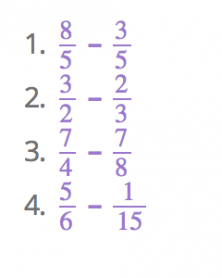Smartick is an advanced online program that teaches kids math and coding in only 15 min. a day

Mar22

# Practice Exercises on Subtracting Fractions

Welcome, again, to the Smartick blog. Today we are going to explain the exercises on subtracting fractions.

The first thing you need to know before going ahead with the exercises is how to calculate the least common multiple of different numbers. The following link will take you to the post where we explain the steps on how to calculate it: Least Common Multiple.

Already know how to find the least common multiple? Let’s move on, then.

Steps to subtract fractions:

### If the fraction has the same denominator:

1. Write the denominator that the fractions have in the final fraction.
2. Subtract the numerators and write the answer in the final fraction.

### Example: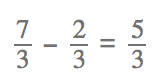### If the fractions have different denominators:

1. Find the least common multiple of the denominators of the fractions.
2. Start writing the new equivalent fractions with the least common multiple as the denominator of these new fractions.
3. The final fraction will have the same denominator that the other fractions had.
4. Subtract the numerators and write the answers down in the final fraction.

### Example: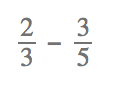The first thing we need to do is find out the least common multiple of the fractions:

LCM (3, 5) = 15

Now, we need to write their equivalent fractions with the denominator as 15: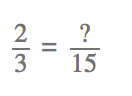In order to solve the numerator number, we’ll have to multiply the numerator (2) by the same number that the denominator was multiplied by.

To go from 3 to 15, we multiplied by 5. That means the numerator needs to be multiplied by 5 as well.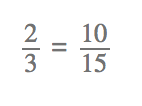And we do the same thing with the other fraction: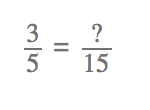We multiplied the denominator by 3, so, the numerator needs to be multiplied by 3, too: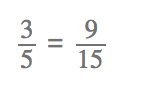Now, we can subtract the two fractions: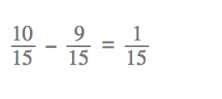### Let’s try another example of subtracting fractions: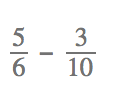The least common multiple of 6 and 10 is 30.

Now, we need to make the equivalent fractions: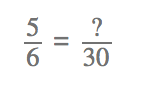We multiplied the denominator by 5, which means we need to multiply the numerator by 5, too: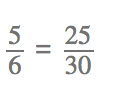And we do the same thing with the other fraction: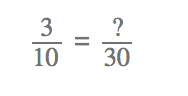We multiplied the denominator by 3, so, we multiply the numerator by 3: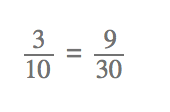Now, we can subtract the fractions: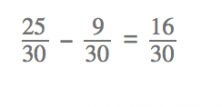Did you learn how to solve exercises on subtracting fractions?

Now, how about you put your skills to the test with the following exercises on subtracting fractions.